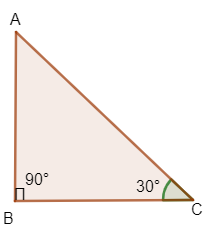Courses
Courses for Kids
Free study material
Free LIVE classes
MoreLIVE
Join Vedantu’s FREE Mastercalss

# If $\sin \theta =\dfrac{3}{5}$, find the values of other trigonometric ratios.Verified
360.9k+ views
Hint:Consider a right angled $\Delta ABC$, right angle at B. Use Pythagoras theorem then find the values of $\cos \theta$, $\tan \theta$ and their reciprocal $\csc \theta$, $\sec \theta$ and $\cot \theta$.

Let us consider a right angled triangle ABC. We know the Pythagoras theorem, also known as Pythagoras theorem; it is a fundamental relation in Euclidean geometry among the 3 sides of a right triangle.It states that the area of the squares whose sides is the hypotenuse is equal to the sum of the areas of the squares on the other two sides.
$A{{B}^{2}}+B{{C}^{2}}=A{{C}^{2}}$
We have been given, $\sin \theta =\dfrac{3}{5}$.
In the triangle ABC, $\angle B=90{}^\circ$
And take $\angle C=\theta$
Here, $\sin \theta =$opposite side/hypotenuse = $\dfrac{AB}{BC}$
$\cos \theta =$Adjacent side/hypotenuse = $\dfrac{BC}{AC}$
Given,$\sin \theta =\dfrac{3}{5}$
AB=3 and AC=5
Using the Pythagoras theorem, $A{{B}^{2}}+B{{C}^{2}}=A{{C}^{2}}$
\begin{align} & {{5}^{2}}={{3}^{2}}+B{{C}^{2}} \\ & \Rightarrow B{{C}^{2}}={{5}^{2}}-{{3}^{2}} \\ & \Rightarrow BC=\sqrt{25-9}=\sqrt{16}=4 \\ & \therefore \cos \theta =\dfrac{4}{5} \\ \end{align}
$\tan \theta =$Opposite side/adjacent side=$\dfrac{AB}{BC}=\dfrac{3}{4}$
$\cos ec\theta =\dfrac{1}{\sin \theta }=\dfrac{1}{\dfrac{3}{5}}=\dfrac{5}{3}$
\begin{align} & \sec \theta =\dfrac{1}{\cos \theta }=\dfrac{1}{\dfrac{4}{5}}=\dfrac{5}{4} \\ & \cot \theta =\dfrac{1}{\tan \theta }=\dfrac{1}{\dfrac{3}{4}}=\dfrac{4}{3} \\ & \therefore \sin \theta =\dfrac{3}{5},\cos \theta =\dfrac{4}{5},\tan \theta =\dfrac{3}{4} \\ & \cos ec\theta =\dfrac{5}{3},\sec \theta =\dfrac{5}{4},\cot \theta =\dfrac{4}{3} \\ \end{align}

Note: There are three types of special right triangle, 30-60-90 triangle, 45-45-90 triangle and Pythagoras triple triangles.

Last updated date: 22nd Sep 2023
Total views: 360.9k
Views today: 7.60k